# Buck Converter | Step Down Chopper

DC-DC converters are also known as Choppers. Here we will have a look at the Step Down Chopper or Buck converter which reduces the input DC voltage to a specified DC output voltage.
A typical Buck converter is shown below.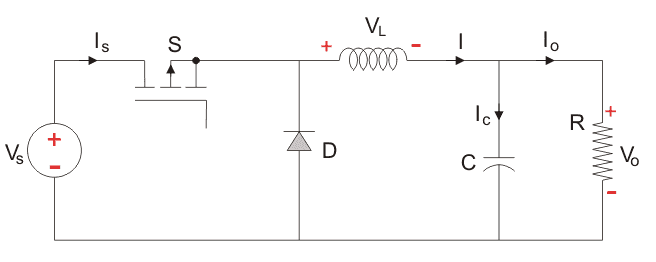The input voltage source is connected to a controllable solid state device which operates as a switch. The solid state device can be a Power MOSFET or IGBT. Thyristors are not used generally for DC-DC converters because to turn off a Thyristor in a DC-DC circuit requires another commutation which involves using another Thyristor, whereas Power MOSFET and IGBT can be turned off by simply having the voltage between the GATE and SOURCE terminals of a Power MOSFET, or, the GATE and COLLECTOR terminals of the IGBT go to zero.
The second switch used is a diode. The switch and the diode are connected to a low-pass LC filter which is appropriately designed to reduce the current and voltage ripples. The load is a purely resistive load.
The input voltage is constant and the current through load is also constant. The load can be seen as current source.

The controlled switch is turned on and off by using Pulse Width Modulation(PWM). PWM can be time based or frequency based. Frequency based modulation has disadvantages like a wide range of frequencies to achieve the desired control of the switch which in turn will give the desired output voltage. This leads to a complicated design for the low-pass LC filter which would be required to handle a large range of frequencies.
Time based Modulation is mostly used for DC-DC converters. It is simple to construct and use. The frequency remains constant in this type of PWM modulation.
The Buck converter has two modes of operation. The first mode is when the switch is on and conducting.

Mode I : Switch is ON, Diode is OFF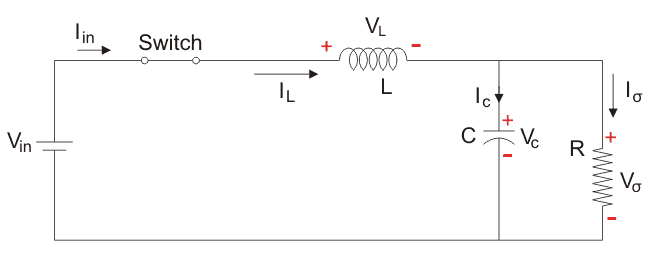The voltage across the capacitance in steady state is equal to the output voltage.
Let us say the switch is on for a time TON and is off for a time TOFF. We define the time period, T, as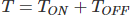and the switching frequency,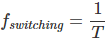Let us now define another term, the duty cycle,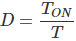Let us analyse the Buck converter in steady state operation for this modeusing KVL.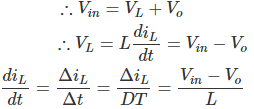Since the switch is closed for a time TON = DT we can say that Δt = DT.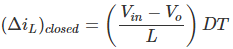While performing the analysis of the Buck converter, we have to keep in mind that

1. The inductor current is continuous and, this is made possible by selecting an appropriate value of L.
2. The inductor current in steady state rises from a value with a positive slope to a maximum value during the ON state and then drops back down to the initial value witha negative slope. Therefore the net change of the inductor current over anyone complete cycle is zero.

Mode II: Switch is OFF, Diode is ON
Here, the energy stored in the inductor is released and is ultimately dissipated in the load resistance, and this helps to maintain the flow of current through the load. But for analysis we keep the original conventions to analyse the circuit using KVL.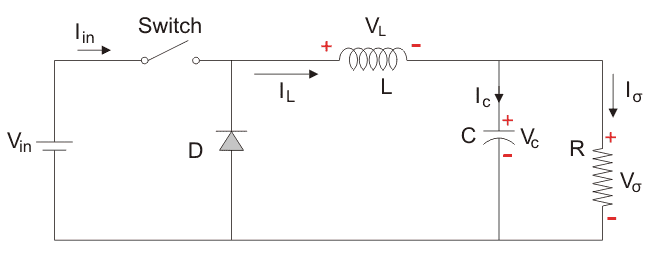Let us now analyse the Buck converter in steady state operation for Mode II using KVL.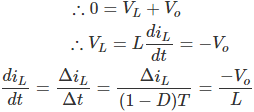Since the switch is open for a time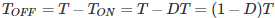we can say that Δt = (1- D)T.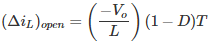It is already established that the net change of the inductor current over anyone complete cycle is zero.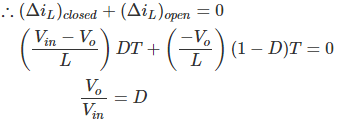A circuit of a Buck converter and its waveforms is shown below.
The inductance, L, is 20mH and the C is 100µF, and the resistive load is 5Ω. The switching frequency is 1 kHz. The input voltage is 100V DC, and the duty cycle is 0.5.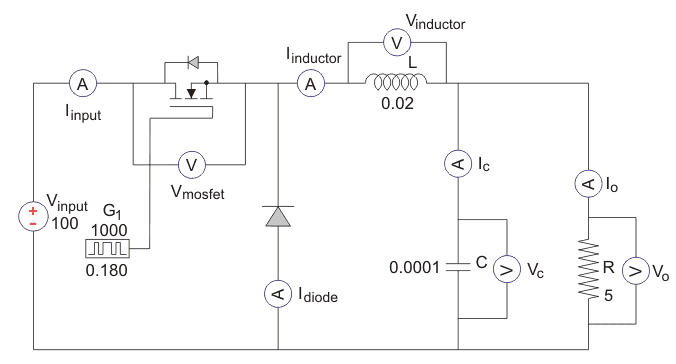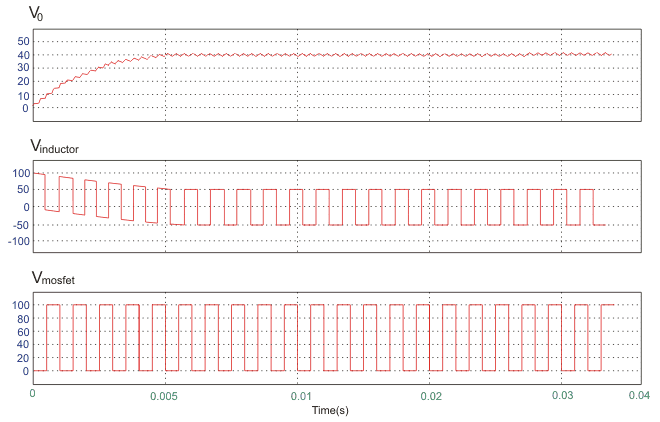The voltage waveforms are as shown above and the current waveforms are as shown in the figure below.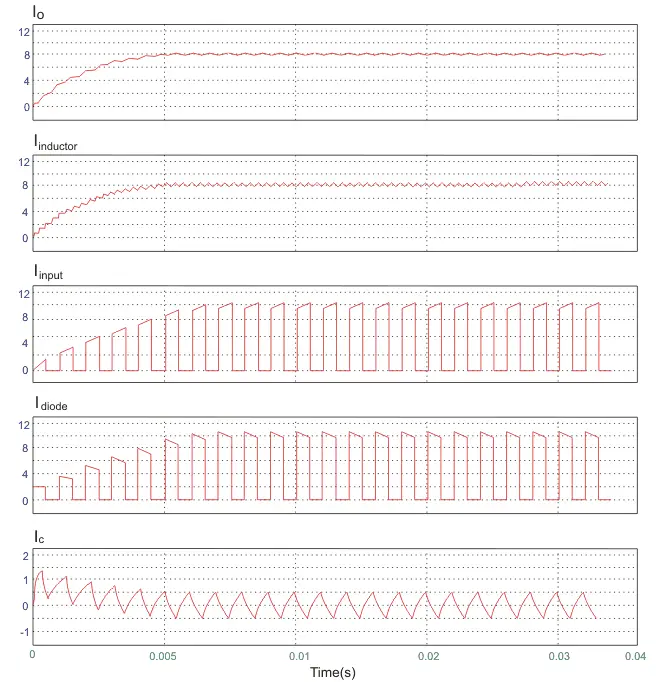Want To Learn Faster? 🎓
Get electrical articles delivered to your inbox every week.
No credit card required—it’s 100% free.